Function Repository Resource:

# IntersectionPlot

Plot two curves and highlight their points of intersection

Contributed by: Wolfram|Alpha Math Team
 ResourceFunction["IntersectionPlot"][{curve1,curve2},{x,lo,hi}] returns a plot of curve1 and curve2 on the domain lo

## Details and Options

ResourceFunction["IntersectionPlot"] takes the options of both Solve and Plot for styling and computation of intersections.
By default, the plot generated by ResourceFunction["IntersectionPlot"] has a legend labelling plot lines according to the curvei.

## Examples

### Basic Examples (1)

Plot the points of intersection of Sin[x] and Cos[x]:

 In:=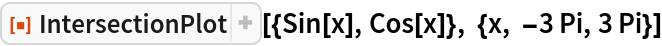Out=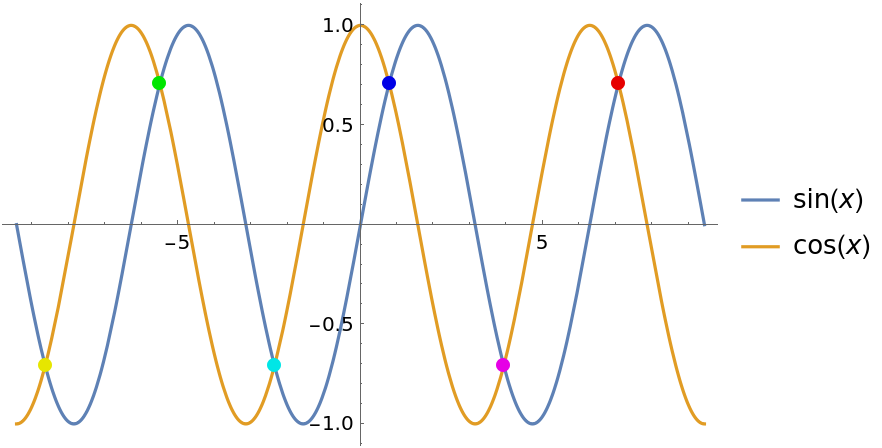### Scope (1)

Use PlotLegends to turn off the legend:

 In:=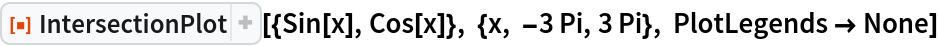Out=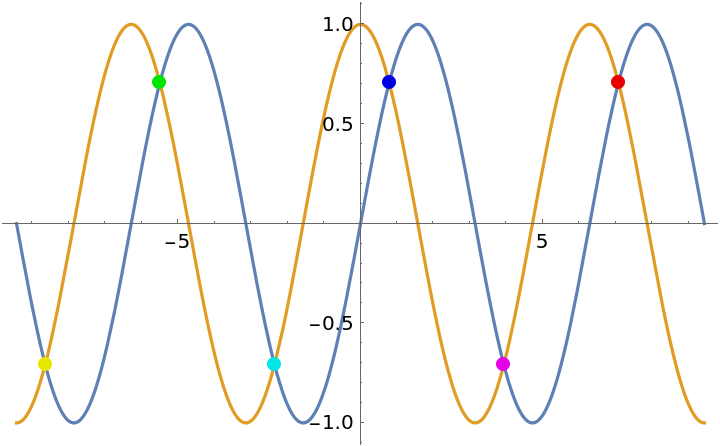### Possible Issues (1)

For curves that intersect infinitely many times, a subset of the points of intersection will be selected:

 In:=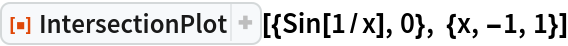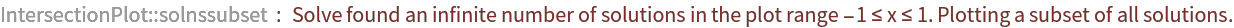Out=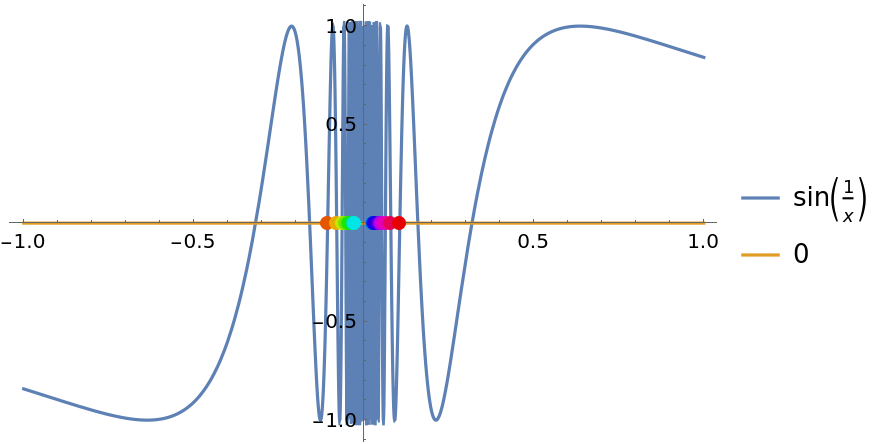## Publisher

Wolfram|Alpha Math Team

## Version History

• 2.0.0 – 23 March 2023
• 1.0.0 – 01 April 2020